7.3. ALGORITHMIC PATTERNS

7.2. COMMUNICATION PATTERNS

In the mpC programs presented in Section 7.1, we used the link declaration to describe the contribution of communication operations in the execution of the parallel algorithm. More specifically, the link declaration in a network type definition specifies:

• the pattern of communication among the abstract processors, meaning a set of communication links over which the abstract processors communicate with each other, and

• the volume of data transferred via each of the communication links.

The communication pattern can be graphically depicted with nodes representing the abstract processors and arcs representing the communication links. The communication pattern described by the link declaration in the definition of the Nbody network type is known as a star (see Figure 7.3). The communication pattern described by the link declaration in the definition of the ParallelAxBT network type is known as a fully connected network (see Figure 7.4).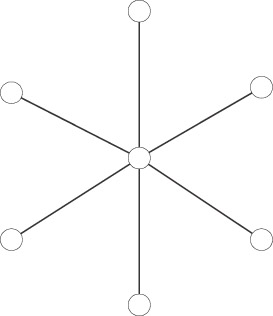Figure 7.3: A star communication pattern.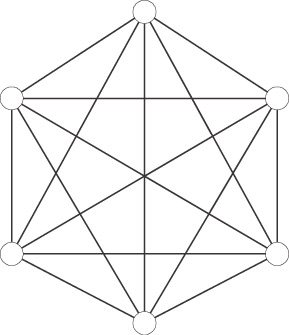Figure 7.4: A fully connected network.

While the communication patterns are very simple, they are widely used in many parallel algorithms. Yet another simple communication pattern is a ring (see Figure 7.5). The next mpC program implements a parallel algorithm using this pattern:

#include <stdio.h> #include <stdlib.h> #include <mpc.h> #define M 100 nettype Ring(int p, double comp, int comm) {    coord I=p;    node { I>=0: bench*comp; };    link    {       I>=0: length*(comm*sizeof(int)) [I]->[(I+1)%p];    };    parent ; }; void [net Ring(n, comp, comm) r] shift(void *a) {    int me, right, left, (*b)[comm];    b=a;    me = I coordof r;    right = (me+1)%n;    left = (me+n -1)%n;    [r:I==right](*b)[] = [r:I==me](*b)[];    [r:I==me](*b)[] = [r:I==left](*b)[]; } int [*]main(int [host]argc, char **[host]argv) {    repl int n;    n = [host]atoi(argv);    {       net Ring(n, 0.000001, M) r;       int [r]a[M];       a[] = I coordof r;       [host]: printf("[+]a[]=%d\n", [+]a[]);       ([([r]n, [r]0.000001, [r]M)r])shift(a);       [host]: printf("[+]a[]=%d\n", [+]a[]);    } }Figure 7.5: A ring communication pattern.

The abstract processors of network r are logically connected into a communication ring. During the program’s execution each abstract processor sends its copy of array a to the neighbor at the right in this ring while receiving its copy of this array from the neighbor at the left. In other words, the program shifts clockwise the distributed array a in this ring.

The network function shift, which performs the shift operation, is not a good programming style example. It is given here only to demonstrate that the mpC language allows the programmer to split a single communication operation into two suboperations, one of which sends data and the other receives it.

For example, execution by each abstract processor of the assignment

[r:I==right](*b)[] = [r:I==me](*b)[];

only results in sending an array pointed by b from this abstract processor to its neighbor at the right. The reason is that variables me and right are not replicated and have different values on different abstract processors. The logical expression I==me will be true on each abstract processor. Therefore all abstract processors will send data during execution of the assignment. The logical expression I==right will be false on each abstract processor, and therefore none of them will receive data during execution of the operation. Similarly execution of assignment

[r:I==me](*b)[] = [r:I==left](*b)[];

by each abstract processor only results in receiving data from its neighbor at the left and storing the data in an array pointed by b.

Although this style of parallel programming can sometimes lead to a more concise and efficient code, it is unsafe and not easy to understand. It is therefore not recommended.

The volume of computation performed by the abstract processors of network r is specified because the contribution of computations into the total execution time is negligibly small compared to that of communications. This informs the compiler to optimize data transfer rather than the computations during the mapping of the abstract processors of network r to the parallel processes of the program.

The next mpC program uses a more complex communication pattern known as a binary tree, meaning a tree with at most two children for each node (see Figure 7.6). In this program the root node of the tree computes some data and broadcasts the data to all other nodes:

#include <stdio.h> #include <stdlib.h> #include <mpc.h> #define M 100 nettype Tree(int p, double comp, int comm) {    coord I=p;    node    {       I==0: bench*comp;       I>0: bench*0.;    };    link    {       I>=0: length*(comm*sizeof(int)) [I]->[2*I+1],                   [I]->[2*I+2];    };    parent ; }; int [*]main(int [host]argc, char **[host]argv) {    repl n; //Number of levels in a binary tree    repl p; //Number of nodes in an n-level perfect binary tree    repl i, j;    n = [host]atoi(argv);    for(i=1, j=2, p=1; i<n; i++, j*=2)       p+=2*i;    {       net Tree(p, 0.000001, M) t;       int [t]a[M];       repl [t]i;       [host]: a[] = p;       [host]: printf("[+]a[]=%d\n", [+]a[]);       for(i=0; i<[t]p/2; i++)       {          [t:I==2*i+1]a[] = [t:I==i]a[];          [t:I==2*i+2]a[] = [t:I==i]a[];       }       [t:I==p-1]: MPC_Printf("[+]a[]=%d\n", [+]a[]);    } }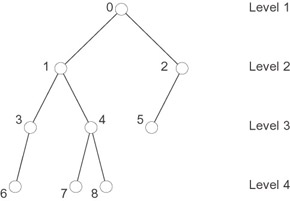Figure 7.6: A four-level binary tree. Node 0 is a root. Nodes 5, 6, 7, and 8 are leaves. Nodes 1, 2, 3, and 4 are interior.

In the program the Tree network type specifies a communication pattern that forms a complete binary tree, that is, an n-level binary tree in which all leaf nodes are at level n or n - 1, level n - 1 is full, and all leaves at level n are toward the left (see Figure 7.7).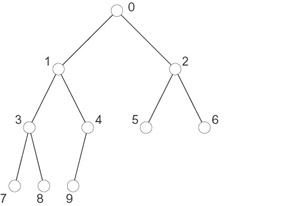Figure 7.7: A four-level complete binary tree. Level 3 is full.

Network t of type Tree is defined in the program so that its communication pattern forms a perfect binary tree. This is a particular case of the complete binary tree in which all levels are full (see Figure 7.8).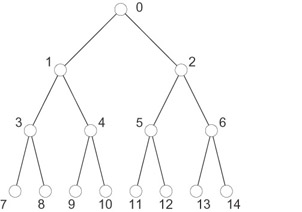Figure 7.8: A four-level perfect binary tree, since all levels are full. An n-level perfect binary tree has 2n - 1 nodes.

The program computes the value of array a for the parent of network t and broadcasts the array to all other abstract processors of this network as follows:

• First the parent, which is the root of the broadcast tree, sends array a to its children.

• Then each interior node of the tree sends array a to its children after it has received this array from its parent.

So far all the communication patterns specified in our programs were static in that while the quantitive characteristics of the communication pattern (e.g., number of nodes and links) could be specified at runtime, the shape of the pattern was always the same. For example, the pattern of communications between the abstract processors of any mpC network of the Nbody type will always form a star no matter what parameters are specified. In general, the mpC language allows dynamic communication patterns.

There are several ways to describe dynamic patterns in mpC. One simple approach is demonstrated by the following network type definition:

nettype Dynamic(int p, int comp, int comm, int pattern) {    coord I=p;    node { I>=0: bench*comp; };    link    {       pattern==STAR: length*comm ->[I];       pattern==RING: length*comm [I]->[(I+1)%p];    };    parent ; };

The link declaration in this definition describes the star or ring communication pattern depending on the parameter pattern.

The mpC language allows the programmer to specify not only regular but also irregular communication patterns. The following example demonstrates how an artibrary irregular coomunication pattern might be specified in mpC:

nettype Irregular(int p, int comp, int comm, int pattern[p][p]) {    coord I=p;    node { I>=0: bench*comp; };    link (J=p)    {       I!=J: length*(comm*pattern[I][J]) [I]->[J];    };    parent ; };

In the definition above, an array parameter pattern is used to specify the presence or absence of communcation between pairs of abstract processors. It is supposed that the value of elements of the array is either 0 or 1. If pattern[I][J] is equal to 0, the link declaration will specify no data transfer from abstract processor I to abstract processor J. If pattern[I][J] is equal to 1, the transfer of comm bytes from abstract processor I to abstract processor J will be specified. Note that as information about the volume of data transfer between abstract processors is calculated and used at runtime, regular communication patterns have no advantage over irregular ones in terms of efficiency.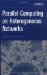Parallel Computing on Heterogeneous Networks (Wiley Series on Parallel and Distributed Computing)
ISBN: B000W7ZQBO
EAN: N/A
Year: 2005
Pages: 95